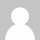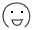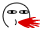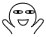# 合理化管理SWAP交互区

```FREE_MEM=\$(free -m | grep - | awk '{print \$3}')
SWAPON=\$(free -m | grep Swap | awk '{print \$2}')
if [ \$FREE_MEM -lt 1536 ] ;then
[ \$SWAPON -ne 0 ]&&swapoff -a
else
[ \$SWAPON -eq 0 ]&&swapon -a
fi```

``````dd bs=1M count=128 if=/dev/zero of=test
dd bs=1M count=128 if=/dev/zero of=test; sync
dd bs=1M count=128 if=/dev/zero of=test conv=fdatasync
dd bs=1M count=128 if=/dev/zero of=test oflag=dsync``````

1.dd bs=1M count=128 if=/dev/zero of=test

2.dd bs=1M count=128 if=/dev/zero of=test; sync

3.dd bs=1M count=128 if=/dev/zero of=test conv=fdatasync

4.dd bs=1M count=128 if=/dev/zero of=test oflag=dsyncTags: , ,

1.都是用的简单的命令，操作swap的不懂

1.MOD回复

@tennfy: 我只是想自动化合理化控制

2.都是技术文章。哎

1.MOD回复

@鬼娃娃: 额

3.LV1回复

我想说这都是些什么。。。

1.MOD回复

@咚门: 没什么，。，，

4.回复

技术文章，单身我却不懂，只能路过看看。1.MOD回复

@海涛:那就看看呗，看着看着就熟了

5.尼玛，一冲动买个了directspace的vps，结果发现自己根本就不会用，傻眼了...

1.MOD回复

@CrazyM:所以说冲动是魔鬼。。。你没先了解vps？

2.@kn007: 折腾一个下午了，学着用putty，然后搞了lnmp一键安装包，然后又绑域名，搭建wordpress，终于把测试站点给搭起来了。头都大了，不过也总算是入了个门。七七，求教啊

3.MOD回复

@CrazyM: 额，我现在是手机，在外面呢。。 你有没有入三亩地的群啊？我们大伙都在，你可以寻求帮助4.@kn007: 哦哦，加了群，回头有时间多交流啊5.MOD回复

@CrazyM: 好